List of Symbols.
 a geometrical dimension, [m] A geometrical dimension, [m] b geometrical dimension, [m] B Biot number, usually hL/k. cp specific heat, [J/kg/K] dsi differential area element on surface Si, [m2]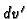differential volume element, [m3] erf (z) error function,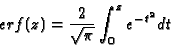f boundary function, units [K] for type 1 boundary, otherwise units  [W/m2] F(r) initial temperature distribution, [K] g volume energy generation,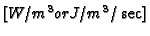G Green's function h heat transfer coefficient, [W/m2/K] H(t) Heaviside unit step function i,j indices J Joule, unit of energy k thermal conductivity [W/m/K] kg kilogram, unit of mass K Kelvin, unit of absolute temperature L geometrical dimension, sometimes body thickness, [m] m fin effect parameter, [m-1]; also meter, unit of length ni outward unit normal vector on surface Si P propagator, function related to the GF q heat flux, [W/m2] r radial coordinate, [m]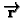position vector, [m] R numbering system designation for radial cylindrical coordinate RS numbering system designation for radial spherical coordinate Si body surface sec second, unit of time t time, [sec] T temperature, [K] x,y,z Cartesian coordinates X, Y, Z numbering system designations for Cartesian coordinates W Watt, a unit of power, equal to one Joule per second.

Greek symbols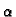thermal diffusivity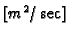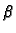eigen value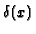Dirac delta function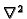Laplacian, spatial derivative in Heat equationsmall parameter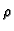density [kg/m3]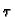time at which the heat pulse is released, [sec]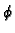azimuthal angle, cylindrical and spherical coordinates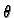polar angle, spherical coordinates